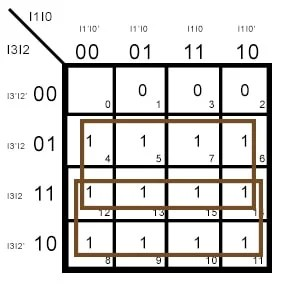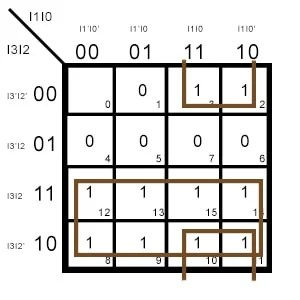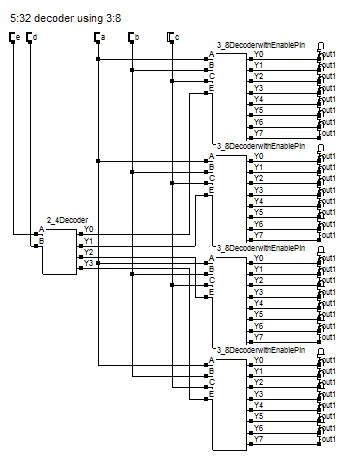View Course Path

# Priority Encoders, Encoders and Decoders – Simple explanation & designing

An n-bit binary number can have 2^n variations. For example, a 2-bit number can have 2^2 variations. 00,01,10 and 11.  This means that we can use an n bit number to store 2 to the power n worth of data. This is essentially the concept of encoding.

To perform this operation, we design a circuit known as an encoder. The circuit’s name is a derivation from its purpose.

A priority encoder is a type of encoder that improves upon the design of a simple encoder.

A decoder is a circuit that undoes the work of an encoder. It’s the opposite of an encoder.

Use the table below to navigate.

Contents

## What is an encoder?

An encoder is a combinational logic circuit that can be used to convert 2^n lines of digital input into n bits of coded binary output. However, in a simple encoder, only one of the inputs is considered to be high out of all the 2^n inputs. In simple terms, an encoder takes in 2^n binary inputs, one at a time, and codes them into n bits of one output code.

## How is an encoder different from a multiplexer?

A multiplexer takes in one of the several inputs and sends it to the output. However, an encoder takes in all the 1s from every input and codes them into an output. The output may be of 2-bits, 3-bits, or 4-bits depending on the number of inputs.

Hence a multiplexer is known as a data selector since it merely selects the data and sends it to the output. An encoder codes the data.

From a hardware perspective, a multiplexer has n inputs and one output. Whereas an encoder has 2^n inputs and n outputs. Multiplexers and encoders have separate applications too. Multiplexers find applications in communication channels. Encoders find applications in compression of information for transmission or storage and for encryption.

## How to design a 4:2 Encoder?

A 4:2 encoder has four inputs and two outputs. By definition, an encoder takes in the high value of every input and gives an n bit output. Essentially, the output that the encoder outputs is the physical address of the line.

What does that mean?

The first input line has a physical address of 1. The first output is 00 (binary) = 0 (decimal). 2^0 = 1, which is the physical address of the first input line. The combination of all the first inputs is 0001 (=1 in decimal).

Similarly, the second output is 01 (binary) = 1 (decimal). 2^1 =2. The combination of all the second inputs is 0010 (=2 in decimal).

Hence in this way, the output of an encoder gives the physical address of the inputs.

### Truth table of 4:2 encoder

 A B C D Y0 Y1 0 0 0 1 0 0 0 0 1 0 0 1 0 1 0 0 1 0 1 0 0 0 1 1

From the truth table of the 4:2 encoder above we have, Y0 = 1 at 0100 or 1000, so,

Y0 = A’BC’D’ + AB’C’D’ = C’D'($A \oplus B$)

Similarly, Y1 = 1 at 0010 or 1000, so,

Y1 = A’B’CD’ + AB’C’D’ = B’D'($A \oplus C$)

Plotting the circuit from the above equations we get the following combinational logic circuit for the 4:2 encoder.

## How to design an 8:3 Encoder?

An 8:3 encoder has eight input lines and three output lines. Let’s write the truth table for the encoder using the information that the encoder gives outputs that are physical addresses of the inputs. For a random example, for an 8-bit input 00001000 (=8 decimal), the 3-bit output should be 011 (=3, 2^3 = 8).

### Truth table of an 8:3 encoder

 A B C D E F G H Y2 Y1 Y0 0 0 0 0 0 0 0 1 0 0 0 0 0 0 0 0 0 1 0 0 0 1 0 0 0 0 0 1 0 0 0 1 0 0 0 0 0 1 0 0 0 0 1 1 0 0 0 1 0 0 0 0 1 0 0 0 0 1 0 0 0 0 0 1 0 1 0 1 0 0 0 0 0 0 1 1 0 1 0 0 0 0 0 0 0 1 1 1

From the above truth table, we have

Y0 = G + E + C + A

Y1 = F + E + B + A

Y2 = A + B + C + D

## What is a Priority Encoder? What is the difference between priority encoders vs encoders?

The encoders we saw above are known as digital or binary encoders. If you observe their truth tables, you will notice that the inputs are a bit peculiar. All the outputs are possible at inputs that have only one high bit. That is, the outputs are present only when exactly one of the input bits is 1. This limits the scope of the data that we can encode. Because if any of the inputs have more than one bit high, the binary encoder gives us an error.

A priority encoder overcomes this disadvantage of the binary encoder. It gives a coded output by assigning a priority to the bits of input. The lower priority bits’ values don’t matter. If the higher priority bit is high, then the priority encoder will generate the output depending on the high bit’s position. The outputs are the same as an encoder, but the range of inputs is higher since the output depends only on the high priority bit. Check out the truth table below to get a clearer picture.

## How to design a 4:2 priority encoder?

A 4:2 priority encoder has four inputs and two outputs like a normal binary encoder. The difference lies in the truth table. For a priority encoder, the output is dependant on the highest priority bit. For example, if I7, I6, and I0 bits of an 8-bit input are high, then the output (111) will be for I7. Let’s write the truth table for a 4:2 priority encoder.

### Truth table of a 4:2 priority encoder

 I3 I2 I1 I0 A B 0 0 0 1 0 0 0 0 1 x 0 1 0 1 x x 1 0 1 x x x 1 1

Here x stands for don’t care condition. It does not matter whether that bit is 0 or 1.

A is high when I2 and I3 are high. Regardless of what the lower bits are. So this means that A is one for

A =1

for 01xx, which when we expand becomes 0100, 0101, 0110, 0111. Thus A is high for all four of these values.

for 1xxx, which when we expand becomes 1000, 1001, 1010, 1011, 1100, 1101, 1110, 1111. Thus A is high for all eight of these values too.

Plotting the K-map for these values, we get for output AA = I2 + I3

and for BB = I3 + I1I2′

From the above equations for A and B, we get the following logic circuit for a 4:2 priority encoder.

## What are the advantages and differences of using a priority encoder rather than a binary encoder?

Binary encoders are good at compressing data. However, the problem with binary encoders is that if more than one of the input bits is high, then it produces an error at the output. Whereas priority encoders were made to overcome this issue. It gives an output by considering only the highest priority bit. Due to this fact and feature, priority encoders are great at handling interrupt requests for a microprocessor.

## What are Decoders?

A decoder is a combinational logic circuit that performs the opposite function of an encoder. Basically, it takes n inputs and gives out 2^n outputs. A decoder is similar to a demultiplexer. However, it has no select inputs.

In a system, if data is encoded using an encoder, it needs a decoder at the other end to decode it. A binary decoder gives output at only one of the output lines depending on the physical address pointed by the inputs.

For example, for the input 10 (n=2 decimal), 2^n = 2^2 = 4 (=0100 binary). Hence the output for 10 will be 0100; that is, the I2 line will be high. Let’s design a 2:4 decoder and understand its truth table.

## How to design a 2:4 Decoder?

A 2:4 decoder has two inputs and four outputs.

### Truth table for a 2:4 decoder

 A B Y3 Y2 Y1 Y0 0 0 0 0 0 1 0 1 0 0 1 0 1 0 0 1 0 0 1 1 1 0 0 0

From the truth table of the 2:4 decoder above we have, Y0 = 1 at A=0 and B=0, so,

Y0 = A’B’

Similarly, Y1 = 1 at A = 0 and B = 1, thus,

Y1 = A’B

Similarly,

Y2 = AB’

and

Y3 = AB

Plotting the circuit from the above equations, we get the following combinational logic circuit for the 2:4 decoder

## How to design a 3:8 Decoder?

A 3:8 decoder has three inputs and eight outputs. Let’s design its truth table and circuit using the logic we saw in the designing of the 2:4 decoder.

### Truth table for a 3:8 decoder

 A B C Y7 Y6 Y5 Y4 Y3 Y2 Y1 Y0 0 0 0 0 0 0 0 0 0 0 1 0 0 1 0 0 0 0 0 0 1 0 0 1 0 0 0 0 0 0 1 0 0 0 1 1 0 0 0 0 1 0 0 0 1 0 0 0 0 0 1 0 0 0 0 1 0 1 0 0 1 0 0 0 0 0 1 1 0 0 1 0 0 0 0 0 0 1 1 1 1 0 0 0 0 0 0 0

From the above truth table

Y0 = A’B’C’

Y1 = A’B’C

Y2 = A’BC’

Y3 = A’BC

Y4 = AB’C’

Y5 = AB’C

Y6 = ABC’

Y7 = ABC

The resulting logic circuit for the 3:8 decoder is as follows.

We can add an enable pin to the chip by just adding it to the AND gates, since AND logic requires all inputs to be high to get a high output. By just adding an enable pin to all the outer AND logic gates, if we want to switch off the decoder,  we can do by grounding the enable pin.

## How to design 4:16 Decoders?

By joining two 3:8 decoders together, we can obtain a 4:16 decoder. We need 16 outputs, which we can easily have as we are using two 3:8 decoders. However, on the input side, we need only four inputs. We have six. So we add the enable pin and make it four inputs on each 3:8 decoder. Joining the eight input pins together, we can get the four inputs we need.

However, one of the pins is an enable pin. And both the 3:8 decoders cannot be active at the same time. Hence, the enable pin to the second decoder is connected with an inverter. So at a time, only one of the decoders is working.